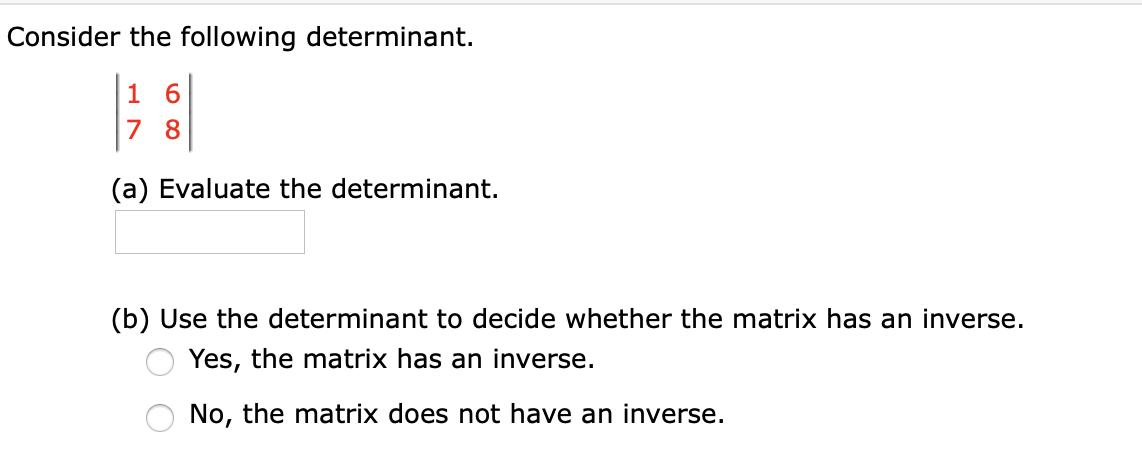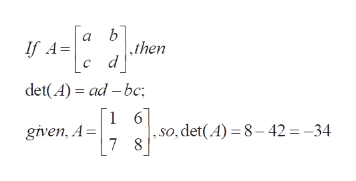# Consider the following determinant.1 67 8(a) Evaluate the determinant.(b) Use the determinant to decide whether the matrix has an inverse.Yes, the matrix has an inverse.No, the matrix does not have an inverse.

Questionhelp_outlineImage TranscriptioncloseConsider the following determinant. 1 6 7 8 (a) Evaluate the determinant. (b) Use the determinant to decide whether the matrix has an inverse. Yes, the matrix has an inverse. No, the matrix does not have an inverse. fullscreen
check_circle

Step 1

To calcuate the value of the determinant, and hence to conclude whether the corresponding matrix is invertible or not

Step 2

a) determinant...help_outlineImage Transcriptionclosea b then If A C det(A) ad -bc _ 1 6] given, A= 7 , s0, det(A) 8-42 -34 fullscreen

### Want to see the full answer?

See Solution

#### Want to see this answer and more?

Solutions are written by subject experts who are available 24/7. Questions are typically answered within 1 hour.*

See Solution
*Response times may vary by subject and question.
Tagged in

### Math